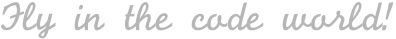| 其他 , 算法&数据结构

• 前言
• 爬楼梯问题
• 递归
• 动态规划
• 矩阵快速幂
• 矩阵乘法
• 快速幂算法
• 通项公式
• 打表
• 总结

## 前言

Ficow 认为这个题目非常棒！原因在于，通过练习掌握这样一个题目，我们就可以学到很多种解法（开拓视野），大致如下：

• 递归
• 动态规划
• 矩阵快速幂
• 通项公式
• 打表

## 爬楼梯问题

1. 1 阶 + 1 阶
2. 2 阶

1. 1 阶 + 1 阶 + 1 阶
2. 1 阶 + 2 阶
3. 2 阶 + 1 阶

## 递归

``````func climbStairsWithRecursion(_ n: Int) -> Int {
var memo = [Int: Int]() // 注意缓存重复的递归状态
func f(_ n: Int) -> Int {
if n < 3 { return n }
if let v = memo[n] { return v }
let v = f(n - 1) + f(n - 2)
memo[n] = v
return v
}
return f(n)
}
``````

• 时间复杂度：O(n)，函数体内没有循环语句，递归调用时没有重复状态，只是利用递归栈进行简单的加法运算。
• 空间复杂度：O(n)，字典缓存重复递归状态占用的空间。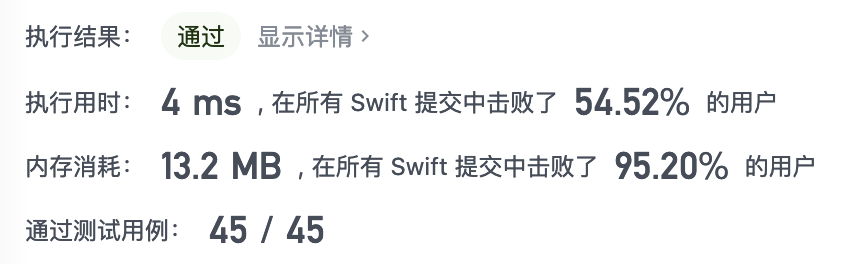## 动态规划

``````let v = f(n - 1) + f(n - 2)
``````

Swift 代码如下：

``````func climbStairsWithDP(_ n: Int) -> Int {
if n < 3 { return n } // edge cases 直接返回
var f = [Int](repeating: 0, count: n + 1)
// base cases
f = 1
f = 2
for i in stride(from: 3, through: n, by: 1) {
f[i] = f[i - 1] + f[i - 2]
}
return f[n]
}
``````

• 时间复杂度：O(n)，函数体内有一个循环语句，从 3 到 n，进行简单的加法运算。
• 空间复杂度：O(n)，动态规划数组占用的空间。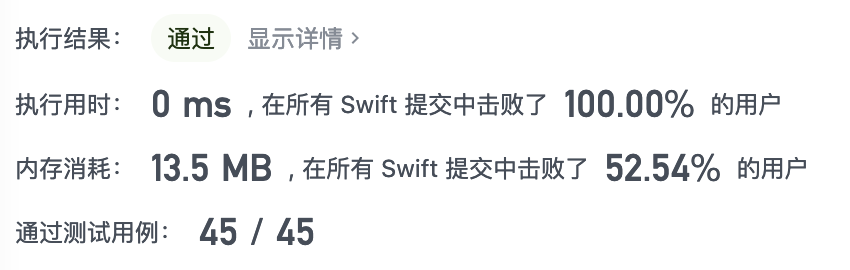### 状态压缩

``````func climbStairsWithCompressedDP(_ n: Int) -> Int {
var a = 0, b = 0, c = 1
for _ in 1...n {
a = b
b = c
c = a + b
}
return c
}
``````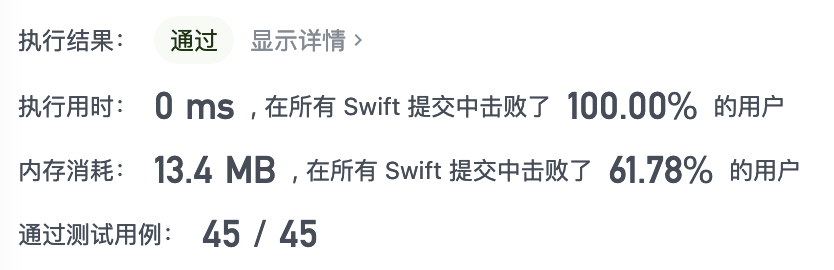## 矩阵快速幂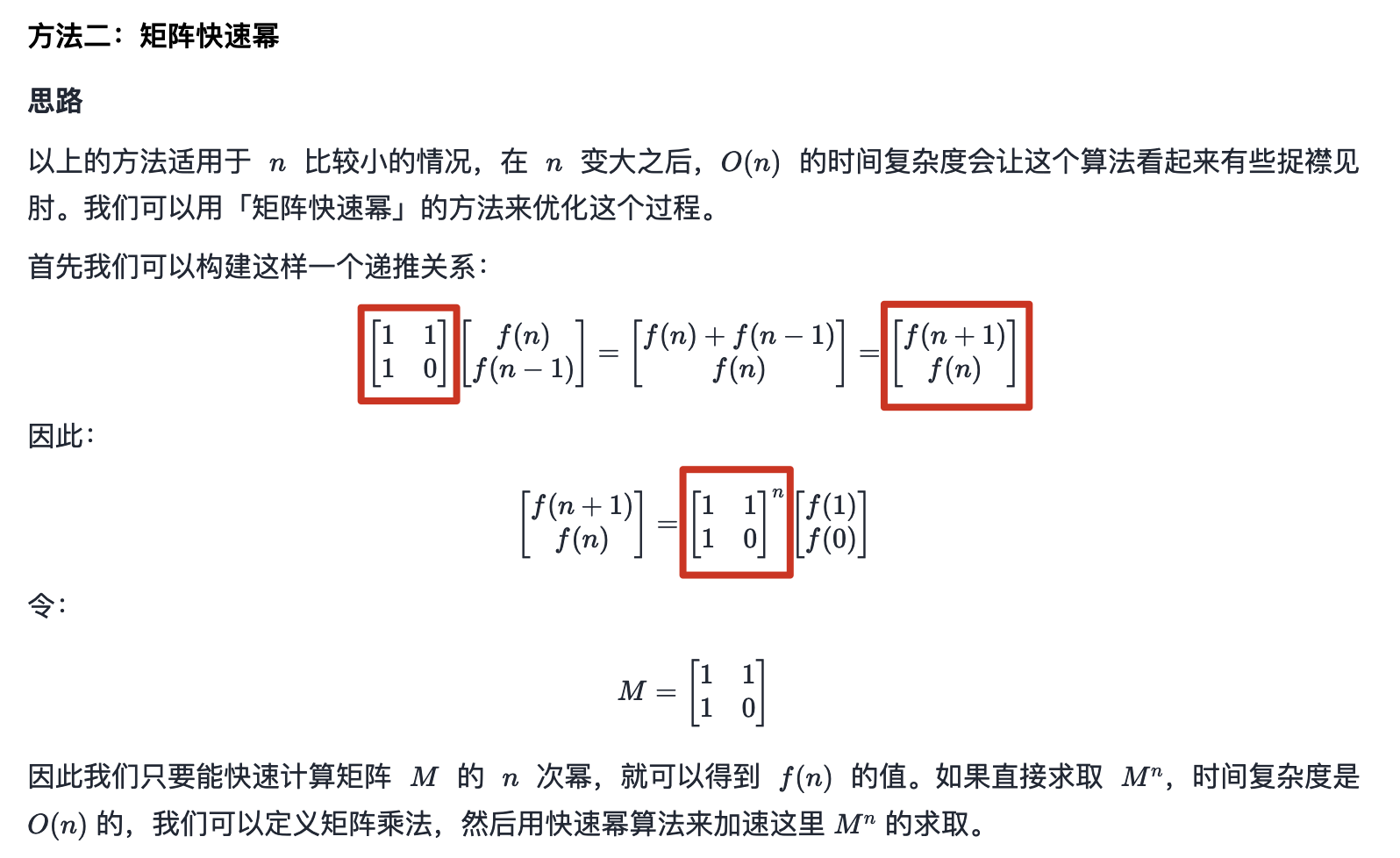### 矩阵运算

#### 矩阵乘法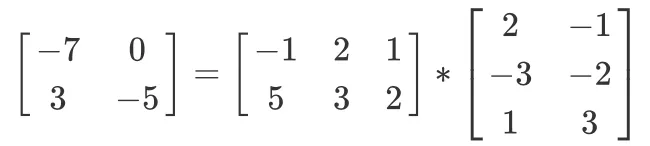#### 构造矩阵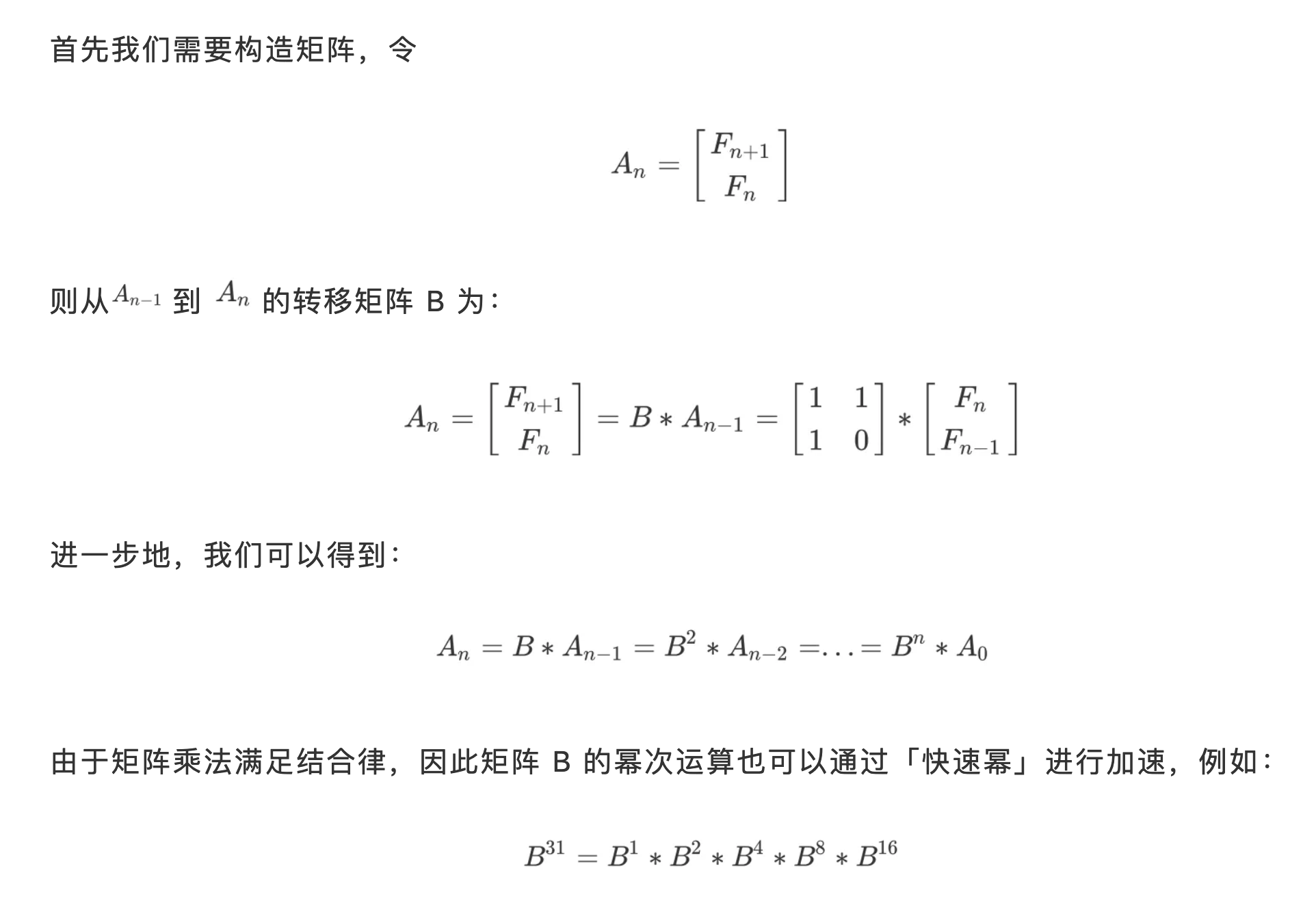#### 转移矩阵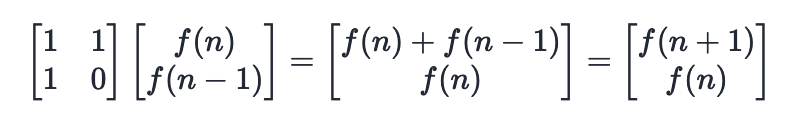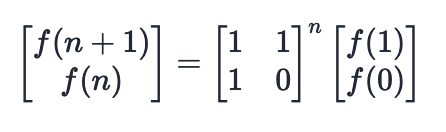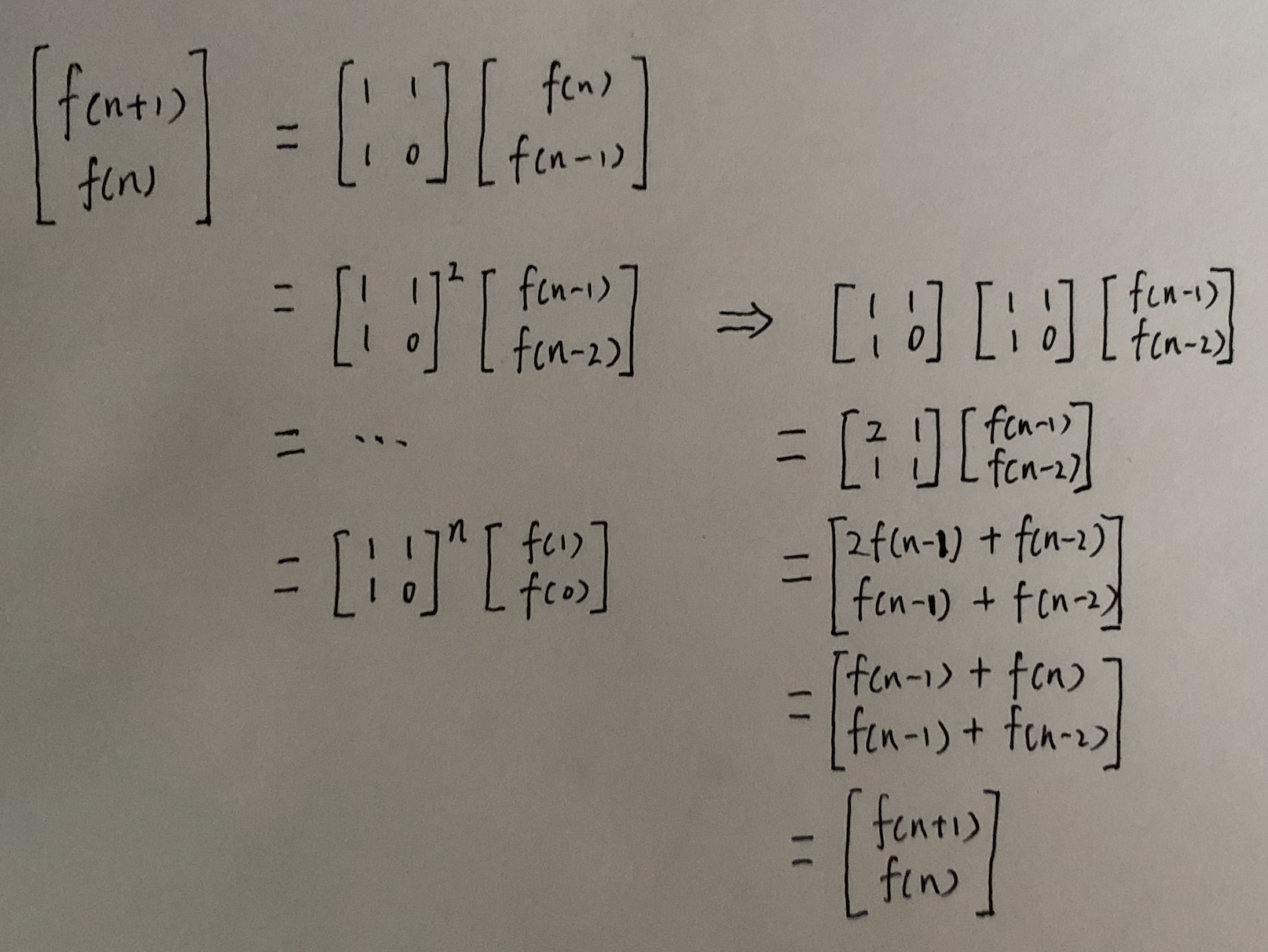### 快速幂算法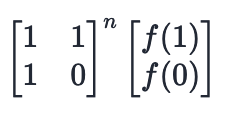#### 递归版本

``````func fastModWithRecursion(_ x: Double, _ n: Int) -> Double {
func mod(x: Double, n: Int) -> Double {
if n == 0 { return 1 }
let y = mod(x: x, n: n >> 1)
return n & 1 == 1 ? y * y * x : y * y
}
return n >= 0 ? mod(x: x, n: n) : 1/mod(x: x, n: -n)
}
``````

#### 迭代版本

``````func fastModWithIteration(_ x: Double, _ n: Int) -> Double {
func f(x: Double, n: Int) -> Double {
var ans = 1 as Double, n = n, xContribute = x
while n > 0 {
if n & 1 == 1 { ans *= xContribute }
xContribute *= xContribute
n >>= 1
}
return ans
}
return n >= 0 ? f(x: x, n: n) : 1/f(x: x, n: -n)
}
``````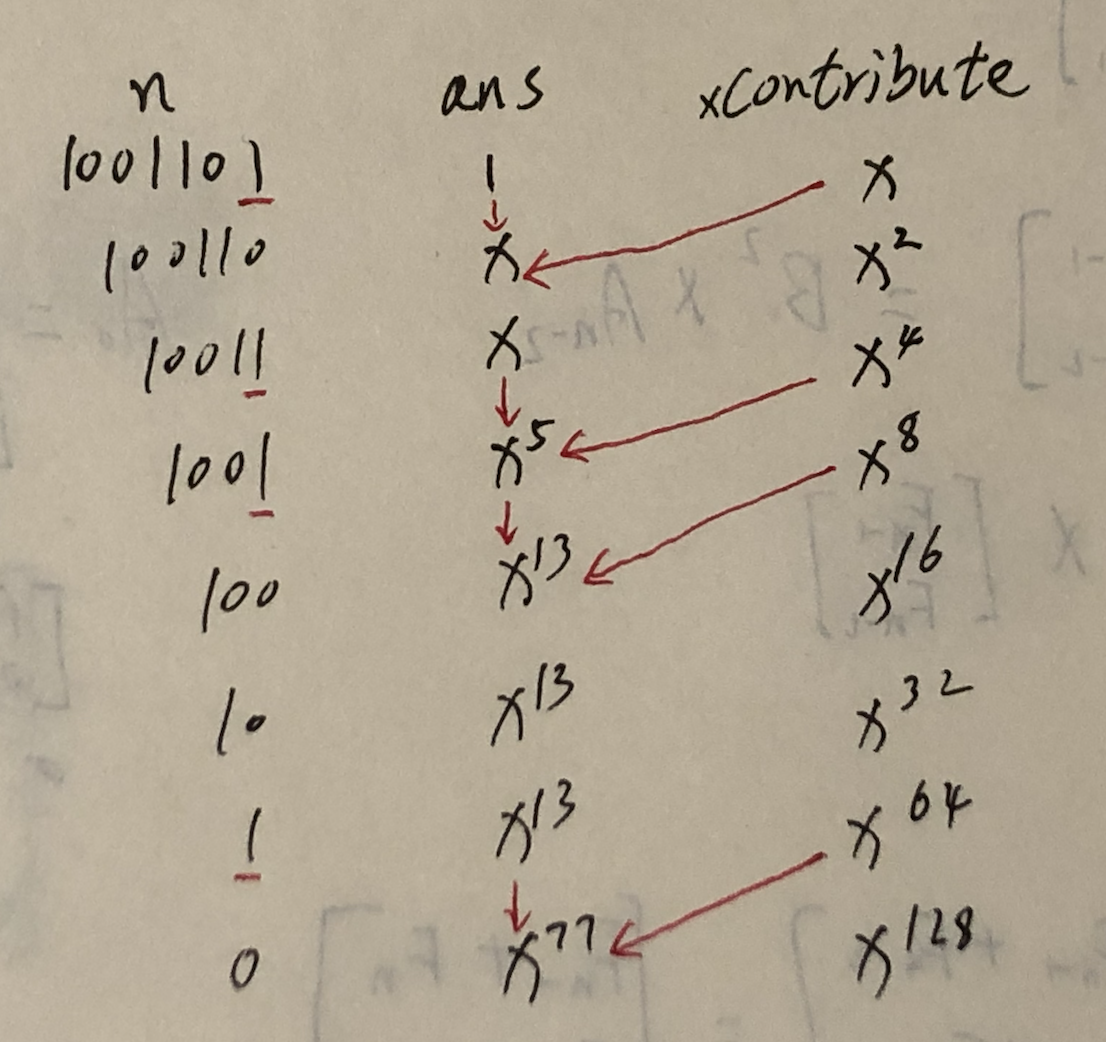### 矩阵快速幂

``````func matrixFastPower(_ n: Int) -> Int {
// 系数矩阵 q
var q = [[1, 1], [1, 0]]
// 让两个矩阵相乘
func multiply(a: [[Int]], b: [[Int]]) -> [[Int]] {
var c = [[Int]](repeating: [Int](repeating: 0, count: 2), count: 2)
for i in 0..<2 {
for j in 0..<2 {
c[i][j] = a[i] * b[j] + a[i] * b[j] // 矩阵乘法
}
}
return c
}
// 快速幂算法的核心代码没有变化
func pow(n: Int) -> [[Int]] {
// 单位矩阵 ret
var ret = [[1, 0], [0, 1]], n = n
while n > 0 {
if n & 1 == 1 {
ret = multiply(a: ret, b: q)
}
n >>= 1 // 二进制右移一位，相当于除以 2 取整
q = multiply(a: q, b: q)
}
return ret
}
let res = pow(n: n)
return res
}
``````

• 如果您还是没明白这部分的知识，您可以考虑结合 【宫水三叶】小姐姐的题解来理解：泰波那切数 —— 一题四解。我个人认为该文章可以有效地帮助您理解 `如何获得以上示例代码中的系数矩阵 q`
• 除此之外，以上示例代码中的 pow 函数内有一个 ret 矩阵，这是一个 单位矩阵。之前的快速幂算法需要用 `ans = 1` 来累乘运算过程中的幂值，这里的单位矩阵就是扮演 ans = 1 的角色。其实，单位矩阵就是矩阵运算里面的 1。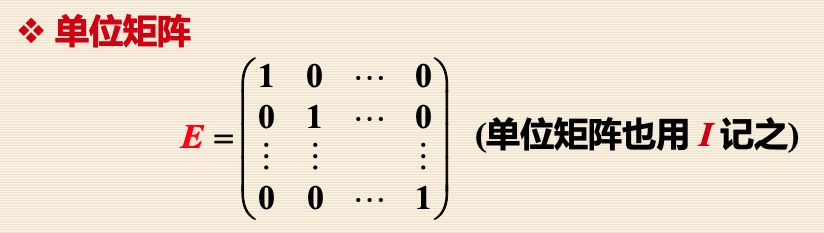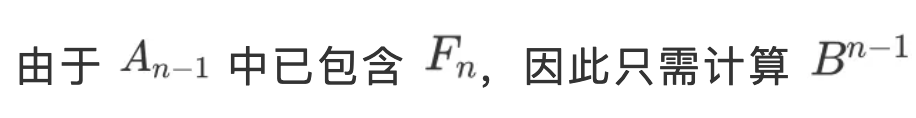## 通项公式

``````func fibonacciFormula(_ n: Int) -> Int {
let sqrt5 = sqrt(5)
let divisor = Double(n + 1)
let fibn: Double = pow((1 + sqrt5) / 2, divisor) - pow((1 - sqrt5) / 2, divisor)
return Int(round(fibn / sqrt5))
}
``````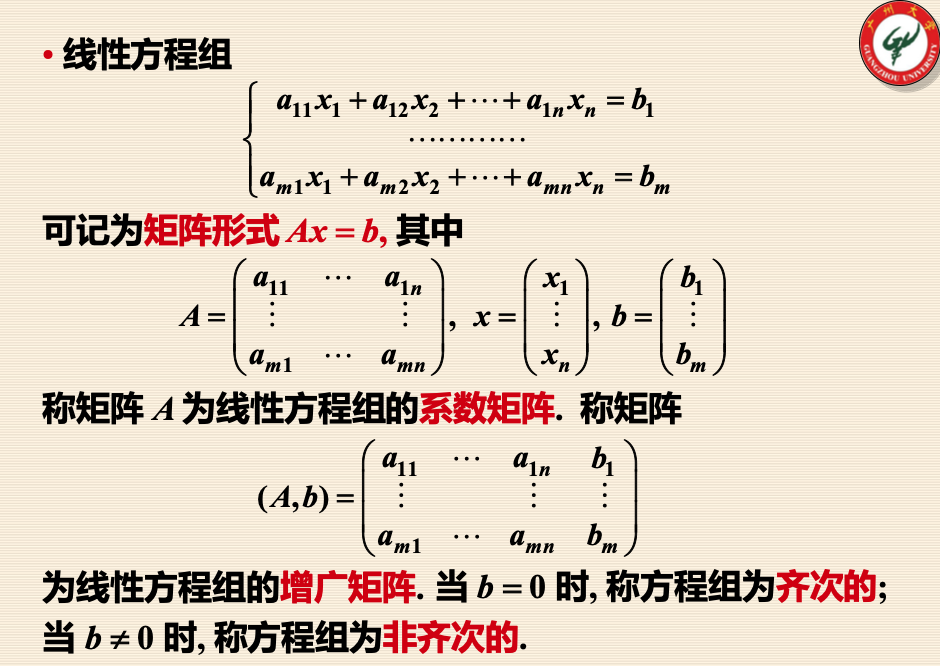## 打表

``````class Solution {
static var values = [Int](repeating: -1, count: 46)

func climbStairs(_ n: Int) -> Int {
if Self.values[n] != -1 { return Self.values[n] }
var a = 0, b = 0, c = 1
for i in 1...45 {
a = b
b = c
c = a + b
Self.values[i] = c
}
return Self.values[n]
}
}
``````

## 总结

#### 评论区(期待你的留言)